# Data ToolReshape data and calculate RMSE, NSE, Pearson Corrolation,...

## What is Data tool?

Assessing the accuracy of predictive models is critical because predictive models have been increasingly used across various disciplines and predictive accuracy determines the quality of resultant predictions. Also, the evaluation of model performance, i.e., to compare model-produced estimates with observed/reliable values, is a fundamental step for model development and use. So, we need an applicable tool for this aim. In this regards, Agrimetsoft has decided to progress a comprehensive Excel add-ins that users can easily sort and reshape their data, then apply the mentioned indices over the sorted data. Before calculating these essential indices with Data Tool, we should reshape the data in a specific format which can able to run them. So, "Data Tool" is an Excel add-ins tool for reshaping and sorting data in excel and convert daily data to monthly or seasonally, and finally calculate efficiency criteria such as Root Mean Square Error, Nash Sutcliffe model Efficiency coefficient, Mean Absolute Error, and other ones. In following this manuscript, you can learn that how can you reshape your data and how can you implement and use different statistical methods for the accuracy of your model and in your model evaluation through Data Tool.

It is necessary to know that validation process commonly includes a criteria definition that relies on mathematical measurements of how well model-produced estimates simulate the observed values. This step is an essential and vital part of the different process of engaging in a project. There are different methods for doing this activity which is called in a different name, such as "Efficiency criteria", "Statistical measures of model performance", "Statistical methods for accuracy", "Model evaluation technique", "Evaluation Statistics", and etc. Several types of research and scholars have problems to calculate different indices, in this regards. So, Agrimetsoft has developed "Data Tool" for solving this issue.

### 1- Reshape Data in Excel

Before the user wants to calculate different efficiency criteria over his data in Data Tool, reshaping data may be necessary. In this case, the Data Tool has two options, namely "Reshape 2Dim" and "Reshape 1Dim". In the first option, the user can reshape data to two dimensions and the second one for reshaping data to one dimension. For better clarification see the help file with an example about Data Tool.

1. Open your Excel file and go to "Data Tool" tab
2. Mark your data and click on "Reshape 1Dim" or "Reshape 2Dim", based on the format of your data. If your data is in one column so click on "Reshape 1Dim" otherwise click on "Reshape 2Dim".
3. If you click on "Reshape 1Dim" so you should enter the number of the columns that you want in the textbox into the window.

Notice: The rows of the data that you mark should be dividable by the number of columns that you enter in the Textbox.

### 2- Convert daily data to monthly or seasonally

Obviously, you can understand the related process from the title of this section, i.e. you can convert your data to the different time scale, in Data Tool. In this case, you have three option for selection in Data Tool, including "Column daily data to month", "Row daily data to month", and "Row monthly to seasonally" (It has an option for a custom season). By converting your data to your desired format, then you can easily select the efficiency criteria and calculate it by Data Tool. Follow these steps:

1. Open your data in Excel file
2. Go to "Data Tool" Tab
4. Click on "Column Daily Data to Month" or "Row Daily Data to Month" based on your data
5. Check the "Add Date Column" and enter Year/Month if you want
6. Check the "Use Leap Year" and enter the First Year if your data has 366 days in leap years.
7. Click on "To Monthly In Rows" Or "To Monthly In Column" based on your request.
8. You can click on the table and press Ctrl+A to select all data and copy them
9. You can click on "Send to new sheet" for sending data to new sheet into your workbook.

### 3- Calculate RMSE (Root Mean Square Error)

The Root Mean Square Error (RMSE) (also called the root mean square deviation, RMSD) is a frequently used measure of the difference between values predicted by a model and the values actually observed from the environment that is being modeled. These individual differences are also called residuals, and the Root Mean Square Error serves to aggregate them into a single measure of predictive power. Root Mean Square Error measures how much error there is between two data sets. In other words, Root Mean Square Error compares a predicted value and an observed or known value.

The Root Mean Square Error is the standard deviation of the residuals (prediction errors). Residuals are a measure of how far from the regression line data points are; Root Mean Square Error is a measure of how to spread out these residuals are. In other words, it tells you how concentrated the data is around the line of best fit. Root mean square error is commonly used in climatology, forecasting, and regression analysis to verify experimental results. The Root Mean Square Error of a model prediction with respect to the estimated variable Xmodel is defined as the square root of the mean squared error:where X-obs has observed values and X-model has modeled values at time/place i

The Root Mean Square Error is always non-negative, and a value of 0 (almost never achieved in practice) would indicate a perfect fit to the data. In general, a lower Root Mean Square Error is better than a higher one. However, comparisons across different types of data would be invalid because the measure is dependent on the scale of the numbers used, so the value of Root Mean Square Error is between 0 to Inf, and it depends on the considered variable.

For example, the amount of precipitation starts from 0 to the maximum number which can occur in a region. The best value of Root Mean Square Error is equal to 0 since in this case, the Root Mean Square Error’s value shows there is not any difference between the observation data and the simulation or model data. The value of Root Mean Square Error is always positive, representing zero in the ideal case. The effect of each error on Root Mean Square Error is proportional to the size of the squared error; thus larger errors have a disproportionately large effect on Root Mean Square Error. Consequently, Root Mean Square Error is sensitive to outliers.

However, the Root Mean Square Error values can be used to distinguish model performance in a calibration period with that of a validation period as well as to compare the individual model performance to that of other predictive models. The Root Mean Square Error measures overall discrepancies between observed and estimated values and the smaller, the better. The Root Mean Square Error has the same units as the variable under analysis.

To adjust for large rare errors, we calculate the Root Mean Square Error with Data Tool. By squaring the errors before we calculate their mean and then taking the square root of the mean, we arrive at a measure of the size of the error that gives more weight to the large but infrequent errors than the mean. We can also compare Root Mean Square Error and Mean Absolute Error to determine whether the forecast contains large but infrequent errors. The larger the difference between Root Mean Square Error and Mean Absolute Error the more inconsistent the error size.

### 4- Calculate NSE (Nash Sutcliffe model Efficiency coefficient)

The Nash-Sutcliffe efficiency (NSE) is a normalized statistic that determines the relative magnitude of the residual variance compared to the measured data variance (Nash and Sutcliffe, 1970). Nash-Sutcliffe efficiency indicates how well the plot of observed versus simulated data fits the 1:1 line. Nash-Sutcliffe efficiency is computed as shown in the following equation: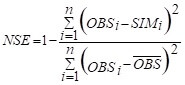Where, OBSi refers to observation data for the desirable variable (such as precipitation, discharge, sediment, runoff, and etc.), SIMi represents the value of simulation or output of the used model for the variable. Nash Sutcliffe efficiencies range from -Inf to 1. Essentially, the closer to 1, the more accurate the model is.

NSE = 1, corresponds to a perfect match of the model to the observed data. NSE = 0, indicates that the model predictions are as accurate as the mean of the observed data, Inf < NSE < 0, indicates that the observed mean is a better predictor than the model.

Nash-Sutcliffe efficiency can be used to quantitatively describe the accuracy of model outputs other than discharge. Nash-Sutcliffe efficiency can be used to describe the predictive accuracy of other models as long as there is observed data to compare the model results with Data Tool. For example, Nash Sutcliffe efficiency has been reported in scientific literature for model simulations of discharge; water quality constituents such as sediment, nitrogen, and phosphorus loading. Other applications are the use of Nash-Sutcliffe coefficients to optimize parameter values of geophysical models, such as models to simulate the coupling between isotope behavior and soil evolution (Legates and McCabe, 1999).

The largest disadvantage of the Nash-Sutcliffe efficiency is the fact that the differences between the observed and predicted values are calculated as squared values. As a result, larger values in a time series are strongly overestimated whereas lower values are neglected (Legates and McCabe, 1999). For the quantification of runoff predictions, this leads to an overestimation of the model performance during peak flows and an underestimation during low flow conditions. Similar to R2, the Nash-Sutcliffe efficiency is not very sensitive to systematic model over- or under prediction especially during low flow periods.

### 5- Calculate Pearson coefficient

Pearson correlation coefficient is the test statistics that measure the statistical relationship, or association, between two continuous variables. It is known as the best method of measuring the association between variables of interest because it is based on the method of covariance. It gives information about the magnitude of the association, or correlation, as well as the direction of the relationship. The Pearson correlation coefficient is a measure of the strength of the linear relationship between two variables.

It is referred to as Pearson's correlation or simply as the correlation coefficient. If the relationship between the variables is not linear, then the correlation coefficient does not adequately represent the strength of the relationship between the variables. Pearson correlation coefficient calculates as follows: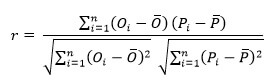where n is the total sets of model data or station-observed data; O and P are the observed data and data model, respectively; and Pi and Oi are the average values of model data and observed data, respectively.

Correlations (Pearson correlation coefficient) are never lower than -1. A correlation of -1 indicates that the data points in a scatter plot lie exactly on a straight descending line; the two variables are perfectly negatively linearly related.

A correlation (Pearson correlation coefficient) of 0 means that two variables don't have any linear relation whatsoever. However, some nonlinear relation may exist between the two variables.

Correlation coefficients (Pearson correlation coefficient) are never higher than 1. A correlation coefficient of 1 means that two variables are perfectly positively linearly related; the dots in a scatter plot lie exactly on a straight ascending line.

The degree of correlation (Pearson correlation coefficient):

1. Perfect: If the Pearson correlation coefficient's value is near +1 and -1, then it said to be a perfect correlation: as one variable increases, the other variable tends to also increase (if positive) or decrease (if negative).

2. High degree of Pearson correlation coefficient: If the coefficient value (Pearson correlation coefficient) lies between +-0.50 and +-1, then it is said to be a strong correlation.

3. A moderate degree of Pearson correlation coefficient: If the value (Pearson correlation coefficient) lies between +- 0.30 and +- 0.49, then it is said to be a medium correlation.

4. Low degree of Pearson correlation coefficient: When the value (Pearson correlation coefficient) lies below +.29, then it is said to be a small correlation.

5. No correlation: When the value is zero.

One of the important questions which arise is related to the Pearson correlation coefficient is: Do the two variables have to be measured in the same units?

No, the two variables can be measured in entirely different units. For example, you could correlate precipitation amount (mm/day) with the percent of humidity in a day (%). Here, the units are completely different; precipitation is measured in mm/day and humidity measured in %. Indeed, the calculations for the Pearson correlation coefficient were designed such that the units of measurement do not affect the calculation. This allows the correlation coefficient to be comparable and not influenced by the units of the variables used.

The following guidelines have been proposed to check the amount of the Pearson correlation coefficient, in summary: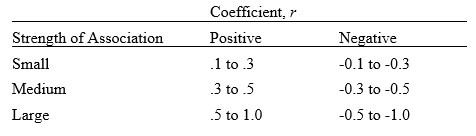### 6- Calculate MBE (Mean Bias Error) coefficient

The Mean bias error is usually not used as a measure of the model error as high individual errors in prediction can also produce a low MBE. Mean bias error is primarily used to estimate the average bias in the model and to decide if any steps need to be taken to correct the model bias. Mean bias error (MBE) captures the average bias in the prediction and is calculated as: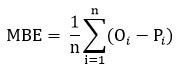Where n is the number of samples, O refers to observation data and P represents the prediction or model data. The long-term performance of a correlation for estimating a value is provided by the Mean bias error. It allows the comparison of actual deviation between the estimated and the measured value for each term. A smaller value of Mean bias error is preferred and ideally, it should be zero. A positive value gives the average amount of overestimation in the calculated value and vice versa. One drawback of this test is that overestimation of an individual observation will cancel underestimation in a separate observation (Dincer et al., 2015).

A positive bias or error in a variable (such as wind speed) represents the data from datasets is overestimated and vice versa, whereas for the variable's direction (such as wind direction) a positive bias represents a clockwise deviation and vice versa. The lower values of errors and considerably higher value of correlation coefficient for the variable and direction are of greater importance.

### 7- Calculate MAE (Mean Absolute Error) coefficient

The simplest measure of forecast accuracy is called Mean Absolute Error (MAE). Mean Absolute Error is simply, as the name suggests, the mean of the absolute errors. The absolute error is the absolute value of the difference between the forecasted value and the actual value. Mean Absolute Error measures accuracy for continuous variables. Mean Absolute Error tells us how big of an error we can expect from the forecast on average. The Mean Absolute Error measures the average magnitude of the errors in a set of predictions, without considering their direction. The Mean Absolute Error is the average over the test sample of the absolute differences between prediction and actual observation where all individual differences have equal weight.

Both Mean Absolute Error and Root Mean Square Error express average model prediction error in units of the variable of interest. The Mean Absolute Error and the Root Mean Square Error can range from 0 to Inf and are indifferent to the direction of errors. They are negatively-oriented scores, which means lower values are better. The value of Mean Absolute Error is calculating by the following equation: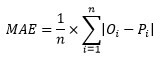n = the number of errors,

Sigma = summation symbol (which means "add them all up"),

|Oi - Pi| = the absolute errors.

### 8- Calculate index of agreement (d)

Willmott (1981) proposed an index of agreement (d) as a standardized measure of the degree of model prediction error which varies between 0 and 1. The index of agreement represents the ratio of the mean square error and the potential error. The agreement value of 1 indicates a perfect match, and 0 indicates no agreement at all. The index of agreement can detect additive and proportional differences in the observed and simulated means and variances; however, d is overly sensitive to extreme values due to the squared differences. The index of the agreement is calculated as follows:Where Oi refers to observation data and Pi shows the prediction or model data. We applied to Mean Absolute Error, Root Mean Square Error, index of agreement, and Nash Sutcliffe efficiency for checking the accuracy of CMIP5 output models i.e. maximum and minimum temperatures and precipitation. For better understanding see the Ullah et al. (2018). Also, for assessing the performance of AgMERRA precipitation data these criteria have been applied by Salehnia et al. (2017).

### 9- Calculate Deviation of runoff Volume

The deviation of runoff volumes Dv, also known as the percentage bias, is perhaps the simplest goodness-fit criterion. The deviation of the runoff volumes is given by WMO (1986), and its value is calculated using the following equation:where Si is the simulated discharge for each time step and Oi is the observed value. N is the total number of values within the period of analysis. For a perfect model, deviation of runoff volumes is equal to zero. The smaller the deviation of runoff volumes value, the better the performance of the model (Gupta et al., 1999). In the case of Dv, there is no need to increase the number of accuracy criteria by using different formulas for a single year or season and for an average of several years or seasons.

### 10- Calculate Deviation of Gain from daily means

In contrast to R2, there are no significant problems in computing the average DG for periods comprising several years. The coefficient of gain from the daily mean, DG, compares model results with daily mean discharge values, which vary throughout the year. DG can vary between 0 and 1, with 0 being a perfect model (WMO, 1986). This coefficient is calculated: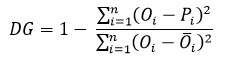where Oi is the measured daily discharge, Pi is the computed daily discharge, and Obar i is the average measured daily discharge.

### 11- Check 12 Distributions

Under construction. We have added it in Data Tool but we are creating the help text and videos.

### 12- CDF Graph

This tool works by Accord Library and we use this class. For using this tool you would select your distribution and mark your data then click on CDF Button. Any column that you select would be a data series. You should not select the headers or any text, you should select just the data.

### 13- PDF Graph

This tool works by Accord Library and we use this class. For using this tool you would select your distribution and mark your data then click on PDF Button. Any column that you select would be a data series. You should not select the headers or any text, you should select just the data.

### 14- BoxPlot

We used BoxPlot in Excel 2016 for this item. It's so simple item and you should select the data series in columns and then click on the BoxPlot button. Then you will the BoxPlot and you can edit the chart. You should not select the headers or any text, you should select just the data.

##### You can calculate several Efficiency coefficients in Online Calculators or
Subscribe to our chanel:

### Reference

1. Dincer I., C. Ozgur Colpan, Onder Kizilkan, et al., 2015. Progress in Clean Energy, Volume 2: Novel Systems and Applications.
2. Gupta, H. V., S. Sorooshian, and P. O. Yapo. 1999. Status of automatic calibration for hydrologic models: Comparison with multilevel expert calibration. J. Hydrol. Eng. 4(2): 135-143.
3. Legates, D.R., McCabe, G.J. (1999). "Evaluating the use of "goodness-of-fit" measures in hydrologic and hydroclimatic model validation". Water Resour. Res. 35: 233-241. doi:10.1029/1998WR900018
4. Nash, J.E., Sutcliffe, J.V., 1970. River flow forecasting through conceptual models, Part I - a discussion of principles. J. Hydrol. 10 (3), 282-290. http://dx.doi.org/10.1016/0022-1694(70)90255-6.
5. Salehnia, N., Alizadeh, A, Sanaeinejad, H, Bannayan, M, Zarrin, A, Hoogenboom, G., 2017. Estimation of meteorological drought indices based on AgMERRA precipitation data and station-observed precipitation data. Journal of Arid Land, 9(6): 798-809. https://link.springer.com/article/10.1007/s40333-017-0070-y
6. Ullah A., Salehnia N., Kolsoumi S., Ahmad A., Khaliq T., 2018. Prediction of efective climate change indicators using statistical downscaling approach and impact assessment on pearl millet (Pennisetum glaucum L.) yield through Genetic Algorithm in Punjab, Pakistan. Ecological Indicators 90: 569-576. https://doi.org/10.1016/j.ecolind.2018.03.053
7. Willmott CJ (1981) On the validation of models. Phys Geogr 2(2):184-194.
8. WMO, 1986. Intercomparison of Models of Snowmelt Runoff. Operational Hydrology Report No. 23, World Meteorological Organization, Geneva.

One Month

8.99\$

Two Months

10.99\$

Three Months

15.99\$

Four Months

20.99\$

Five Months

24.99\$

Six Months

27.99\$